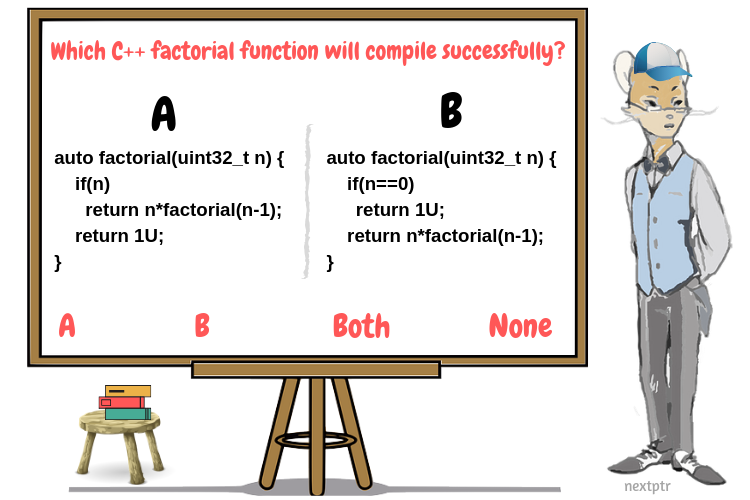# Type deduction of a recursive function that returns autoA C++11 compiler can deduce the return type of a lambda expression that has no explicit return type (or has auto return type):

`````` /* Define and invoke a lambda that returns a double */
auto lret = [] () { return 10.5; }();

// 'lret' is deduced as double
std::cout << typeid(lret).name() << "\n"; // logs 'd' or 'double'
``````

The C++14 standard extended that feature to regular functions:

``````// Return type is deduced to int
auto func() { return 10; }

auto fret = func();

// 'fret' is deduced as int
std::cout << typeid(fret).name() << "\n"; // logs 'i' or 'int'
``````

However, there are some conditions when the compiler cannot deduce the return type of a function. One of those restrictions is on the implementation of recursive functions. These are 2 equivalent implementations of the recursive factorial function:

A

``````auto factorial(uint32_t n) {
if(n)
return n*factorial(n-1);
return 1U;
}
``````

B

``````auto factorial(uint32_t n) {
if(n == 0)
return 1U;
return n*factorial(n-1);
}
``````

Select which of A and/or B can be successfully compiled (Check `Explanations` to learn about correct answer):